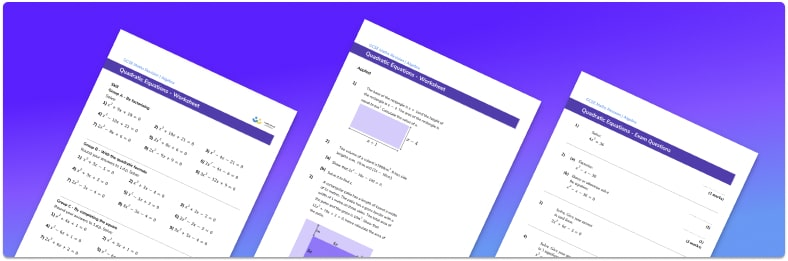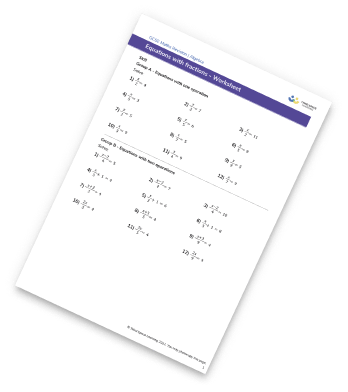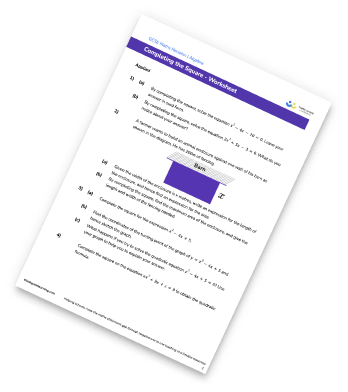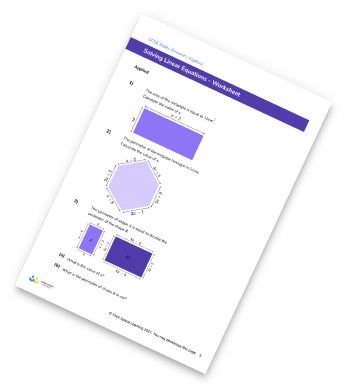• Section 1 of the quadratic equations worksheet contains 20+ skills-based quadratic equations questions, in 3 groups to support differentiation
• Section 2 contains 3 applied questions with a mix of worded problems and deeper problem solving questions
• Section 3 contains 3 foundation and higher level GCSE exam style questions to help practise a variety of methods for solving quadratic equations
• Answers and a mark scheme for all quadratic equations questions are provided
• Questions follow variation theory with plenty of opportunities for students to work independently at their own level
• All questions created by fully qualified expert secondary maths teachers
• Suitable for GCSE maths revision for AQA, OCR and Edexcel exam boards

• This field is for validation purposes and should be left unchanged.

You can unsubscribe at any time (each email we send will contain an easy way to unsubscribe). To find out more about how we use your data, see our privacy policy.

### Quadratic equations at a glance

Quadratic equations contain variables that are raised to a power no higher than two. These can be solved using a variety of methods and there are usually two solutions.

If there is a single squared variable in the quadratic equation it can be solved by rearranging the equation to put the unknown on one side of the equals sign and all the other terms on the other side. If the equation is more complex and contains a squared term and the linear term we can use a variety of methods to solve it.

One method is factorising quadratics. After rearranging the equation so that the right hand side is equal to 0, the quadratic expression on the left hand side of the equals sign is factorised so that it is written as a product of two factors. Then each factor is considered in turn to be equal to zero and the solution is found.

Another method we can use to solve quadratic equations is using the quadratic formula. Here the coefficients of the different terms are substituted into the formula and the solutions are calculated. The solutions can be left as surds (with square roots), or written as decimals as required. We can work out the number of solutions a quadratic equation has by using the discriminant.

Completing the square can also be used to solve a quadratic equation; this method can help to identify the turning points of the quadratic graph produced from the equation.

Looking forward, students can progress with more solving equations worksheets and to additional algebra worksheets, for example a factorising worksheet, or a simultaneous equations worksheet.For more teaching and learning support on Algebra our GCSE maths lessons provide step by step support for all GCSE maths concepts.

## Related worksheets

Forming And Solving Equations WorksheetSolving Equations with Fractions WorksheetCompleting The Square WorksheetLinear Equations Worksheet## Do you have KS4 students who need more focused attention to succeed at GCSE?There will be students in your class who require individual attention to help them succeed in their maths GCSEs. In a class of 30, it’s not always easy to provide.

Help your students feel confident with exam-style questions and the strategies they’ll need to answer them correctly with our dedicated GCSE maths revision programme.

Lessons are selected to provide support where each student needs it most, and specially-trained GCSE maths tutors adapt the pitch and pace of each lesson. This ensures a personalised revision programme that raises grades and boosts confidence.

Find out more